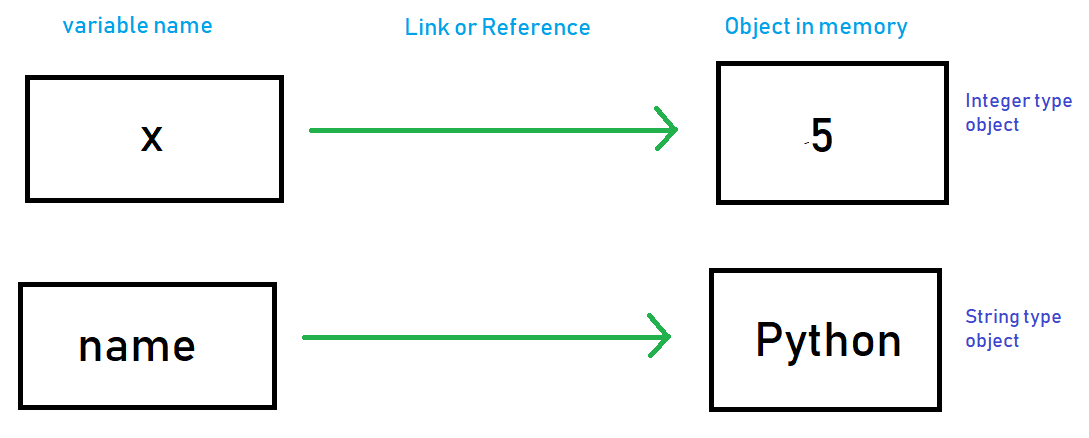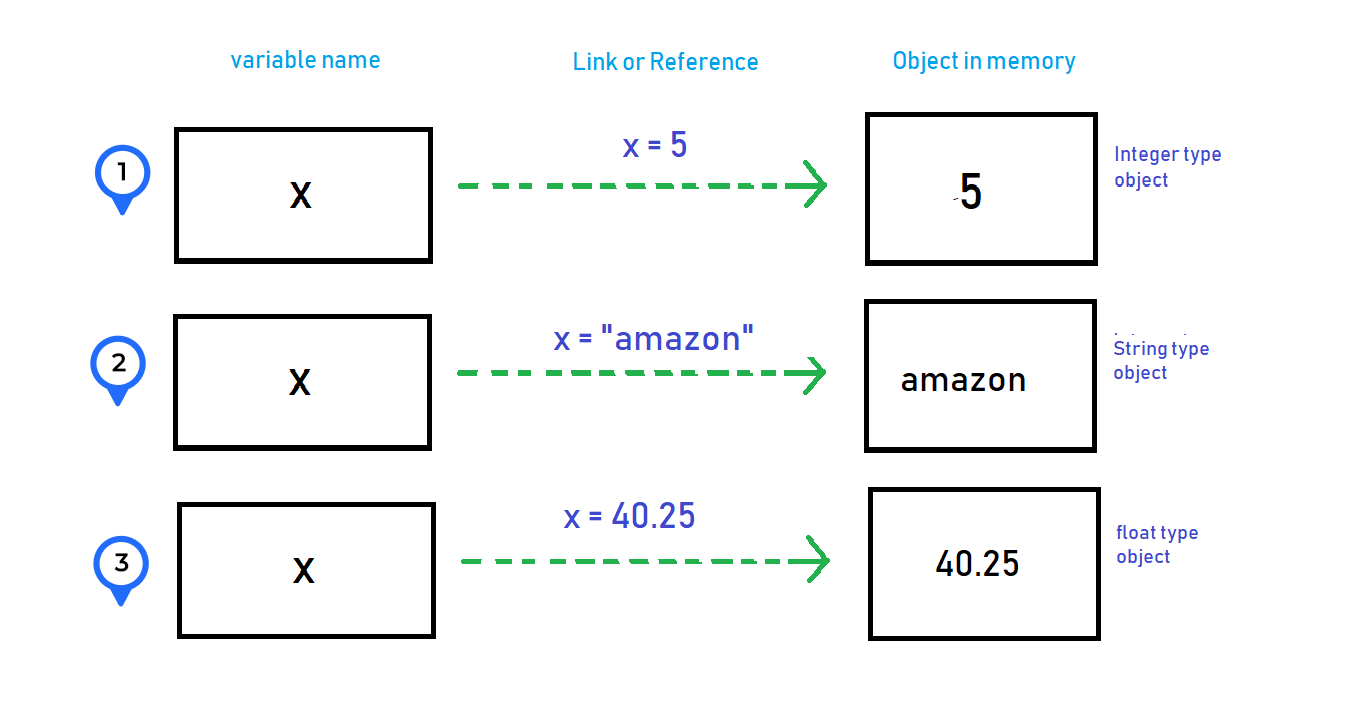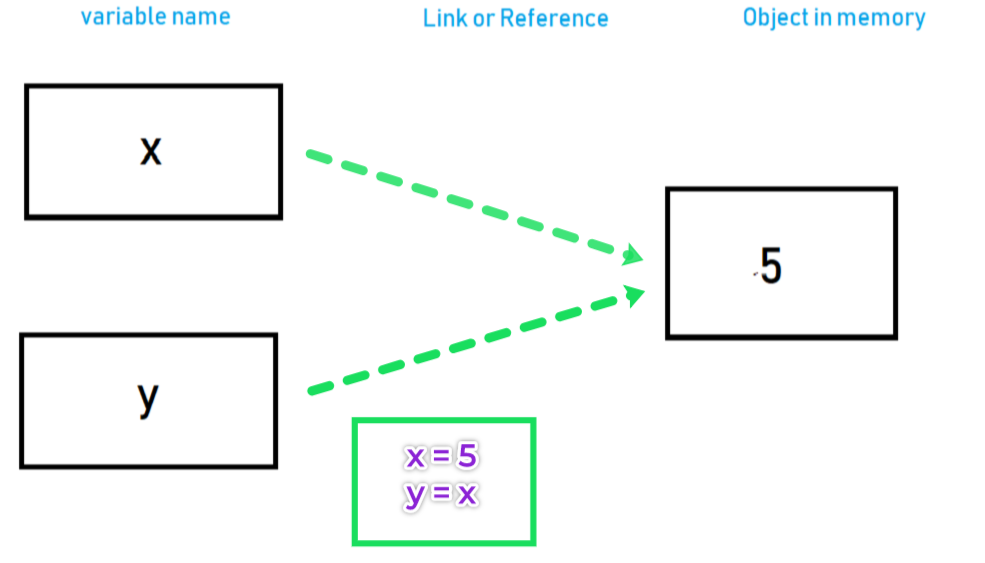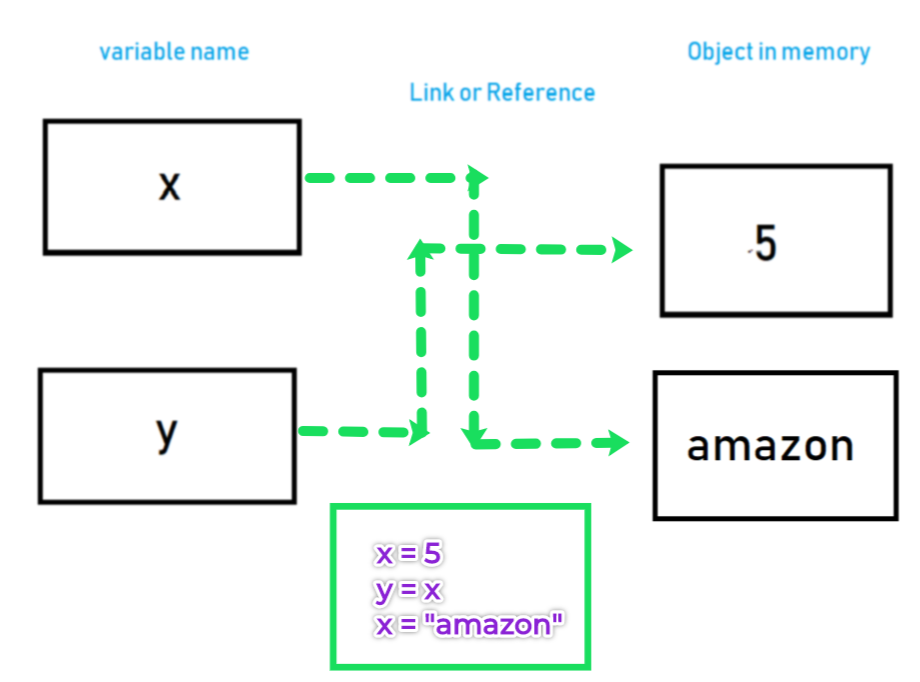# Variables And Keywords In Python

## Variables or identifiers in python

In general, the variables are the names that hold a data stored in the memory and it should have an unique name known as identifier.

## Identifier names and rules

Identifiers in python are the names which are used to identify an entiry like variables, functions, classes etc.

``my_var = 10``

Here "my_var" is the identifier or variable name that holds 10 value.

Rules:

• An identifier can start with a letter A to Z or a to z or an underscore (_) followed by zero or more letters, underscores and digits (0 to 9).
• A identifier can not start with number.( example - 1name is invalid ). But numbers can be used anywhere after first place (na1me, name1)
• Key words can not be used as identifier. (check next section)
• Special symbols like  @, \$, #, % , !etc. can not be used in identifier.(n@name is invalid)

## What are the Keywords in python?

Keywords are the reserved words in Python.

Inside python these words have its own meaning and usability.

The follwoing are the keywords in python

``````'False', 'None', 'True', 'and', 'as', 'assert', 'break', 'class', 'continue', 'def',
'del', 'elif','else', 'except', 'finally', 'for', 'from', 'global', 'if', 'import', 'in', 'is',
'lambda', 'nonlocal', 'not', 'or', 'pass', 'raise', 'return', 'try', 'while', 'with', 'yield'
``````

Remember these key words are specific to a particular version of python. Some keywords may be different in different versions

## Declaring and assigning value to a variable.

In Python you do not need to declare a variable type, because the types are determined automatically at runtime when we assign a value to it. This is know an dynamically typed. (Python is a dynamically typed language)
The data what we assign to a variable takes the form of objects. i.e. either built-in objects that Python provides, or objects we create using Python classes.
Objects are just pieces of memory, with values (and sets of associated operations).
Check the following example.

``````x = 5
name = "Python"
salary =  60.50
``````

Here xname and salary are the variable names on the left side of "=" on each statement and on the right side are its values that are assigned.
Note that we have not pre-declared the variable names and its type before assigning values (generally required in languages like C, C++ , Java).

5, "Python" and 60.50 are nothing but the literals that creates the objects of a particular type. So "x" holds integer type data, "name" -> string and "salary" -> float type of data with 5, "Python" and 60.50 values respectively within it.

Python does this process of determining type automatically at the run time, when we assigned data objects or values (5, "Python" and 60.50 ) which are known as built-in object types or standard data types in Python.

Note *

If a variable is not “defined” (assigned a value), trying to use it will give you an error:

``````print(N) # N is not defined
``````

Traceback (most recent call last):
File "D:/pythoneasy/variables.py", line 1, in <module> print(N) NameError: name 'N' is not defined

## How does Python process a variable

Everything we process in Python programs is a kind of object.
Object is a distinct piece of memory.
Each time you generate a new value in your program by running an expression like above, Python creates a new object ("5" in this case) to represent that value for you.
When we write the expression `x = 5`, Python interpreter does the following steps

1. It creates an object to represent 5 in the memory (the type determined automatically which is integer here).
2. It creates a variable name x.
3. It creates links between the variable x to object 5 which known as reference.## Changing value of variables

Check the below example

``````x = 5   # It's an integer
x = "amazon" # It's a string
x = 40.25   # It's a float
``````
1. We assign 5 to "X" it becomes integer type (it refers to an integer type object 5 in actual).
2. When we reassign "amazon" which a string type of object to "x" it becomes a string type. Eventually in this step Python freed the memory that object "5" holds. This is a part of automatic garbage collection by python.
3. The we reassign 40.25 a float value to "x" and it becomes float type and Python frees the memory that "amazon" holds.

The type changes as we keep on assigning different type of object to it and the variable keep changing its reference to that object in memory.
Therefore the names or variables names does keep the type but the type lives with objects ( 5, "amazon", 40.25), so to whomever these gets assigned the names become that particular type.## Shared Reference

Check this example

``````x = 5
y = x
``````

We assign 5 to "x", so it refers/links to an object "5" in memory.
When we assign "y" to "x" both the "x" and "y" refers to same object of "5" which is known as shared reference.(Check the image below)``````x = 5
y = x
x = "amazon"
``````

`x = "amazon"` - here we reassign a new string value to "x", so a new object with string "amazon" is created and then Python sets "x" to refer it.
Now this time "y" value is not changed and it still refers to the old integer object "5" in the memory (Check the image below)## Multiple Assignment

We can assign multiple values to multiple variables in a single line.
Example:

``````x, y, z = 10, 20, "amazon"
print(x) # 10
print(y) # 20
print(z) # "amazon"
``````

10
20
amazon

We can assign same value to multiple variables at a time in single line.
Example:

``````x = y = z = 10
print(x)
print(y)
print(z)
``````

10
10
10